You are currently offline. Some features of the site may not work correctly.

# Domatic number

Known as: Domat, Domatic number problem, Domatic partition
The domatic number is the maximum size of a domatic partition, that is, the maximum number of disjoint dominating sets. The graph in the figure has… Expand
Wikipedia

## Papers overview

Semantic Scholar uses AI to extract papers important to this topic.
2007
2007
A dominating set \(S\) in a graph \(G\) is a tree dominating set of \(G\) if the subgraph induced by \(S\) is a tree. The tree… Expand
Is this relevant?
Highly Cited
2005
Highly Cited
2005
• 19th IEEE International Parallel and Distributed…
• 2005
• Corpus ID: 8853272
We investigate the problem of maximizing the lifetime of wireless ad hoc and sensor networks. Being battery powered, nodes in… Expand
•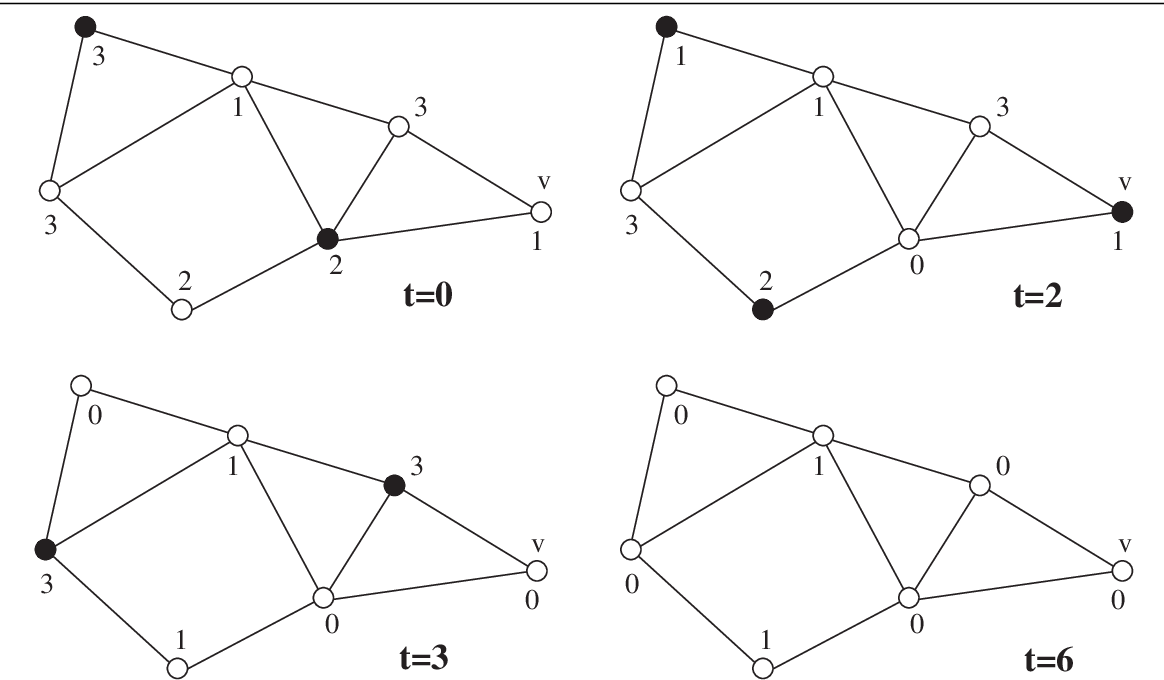Is this relevant?
2005
2005
• Discret. Appl. Math.
• 2005
• Corpus ID: 39476620
Let G be a finite and simple graph with the vertex set V(G), and let f : V(G) → {-1, 1} be a two-valued function. If ∑x ∈ N[v]f(x… Expand
Is this relevant?
Highly Cited
2005
Highly Cited
2005
• ICALP
• 2005
• Corpus ID: 1379989
Davis-Putnam-style exponential-time backtracking algorithms are the most common algorithms used for finding exact solutions of NP… Expand
Is this relevant?
2004
2004
• Ars Comb.
• 2004
• Corpus ID: 15254982
The domatic number of a graph G is the maximum number of dominating sets into which the vertex set of G can be partitioned. We… Expand
Is this relevant?
Highly Cited
2002
Highly Cited
2002
• SIAM J. Comput.
• 2002
• Corpus ID: 118502711
A set of vertices in a graph is a dominating set if every vertex outside the set has a neighbor in the set. The domatic number… Expand
Is this relevant?
Highly Cited
2000
Highly Cited
2000
• STOC '00
• 2000
• Corpus ID: 4645057
A set of vertices in a graph is a dominating set if every vertex outside the set has a neighbor in the set. The domatic number… Expand
•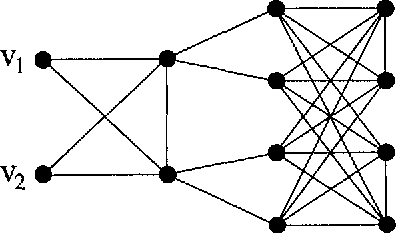Is this relevant?
Highly Cited
1994
Highly Cited
1994
• J. Telle
• Nord. J. Comput.
• 1994
• Corpus ID: 5539691
Many graph parameters are the optimal value of an objective function over selected subsets S of vertices with some constraint on… Expand
•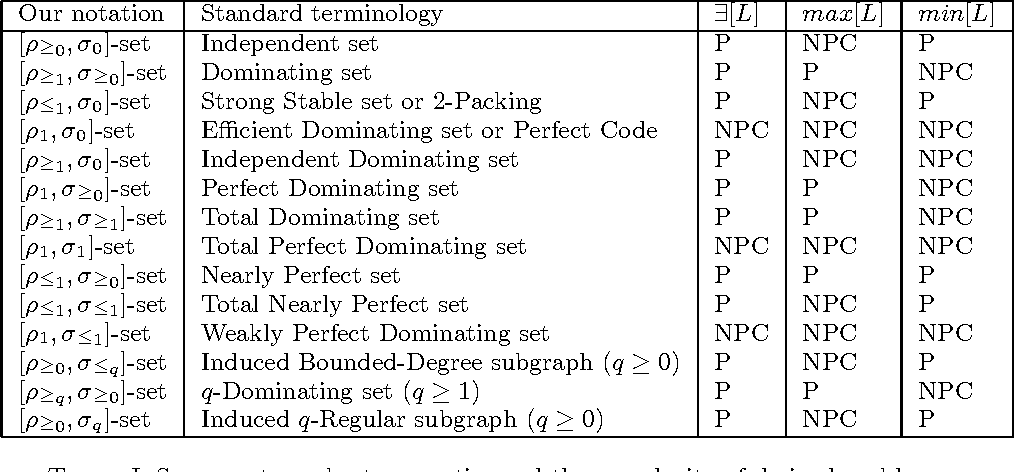•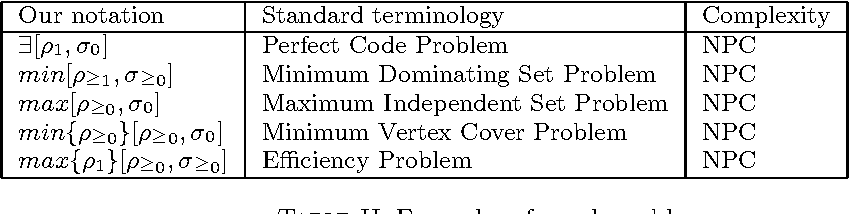•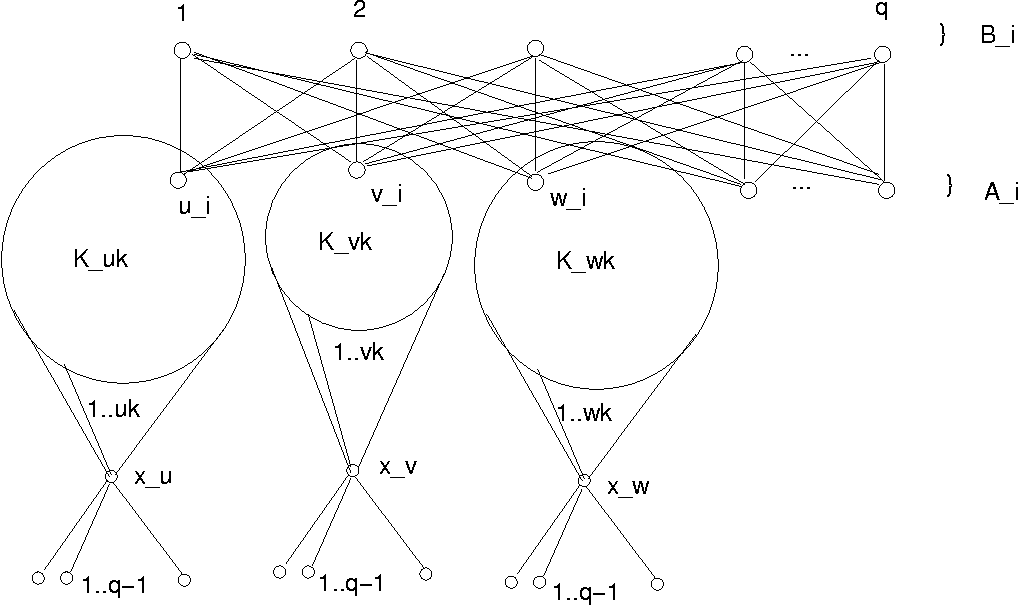•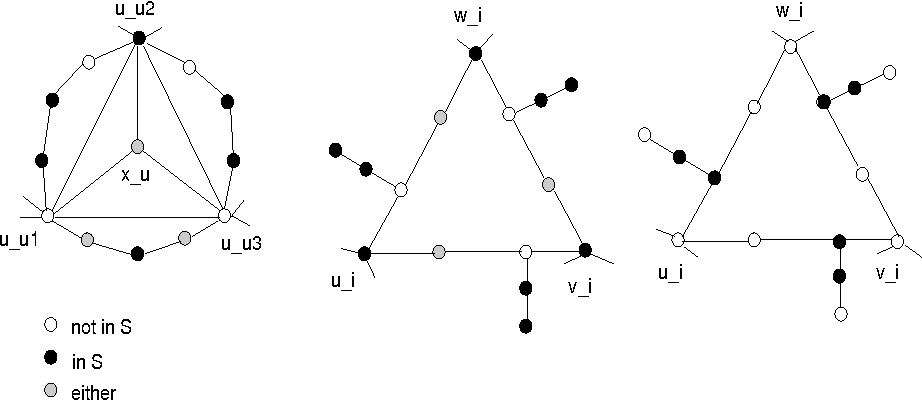•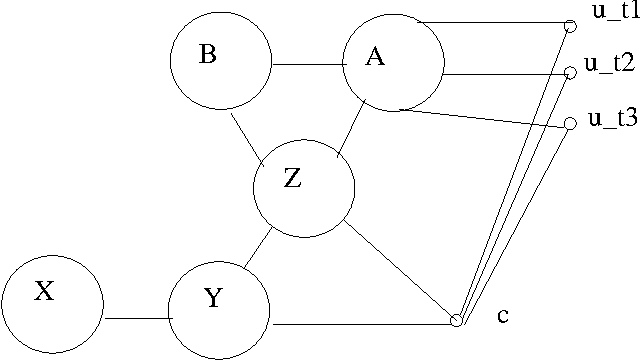Is this relevant?
1988
1988
A dominatmg set of a graph G is a subset S of the nodes such that every uode of G is either in S or adjacent to some node in S… Expand
•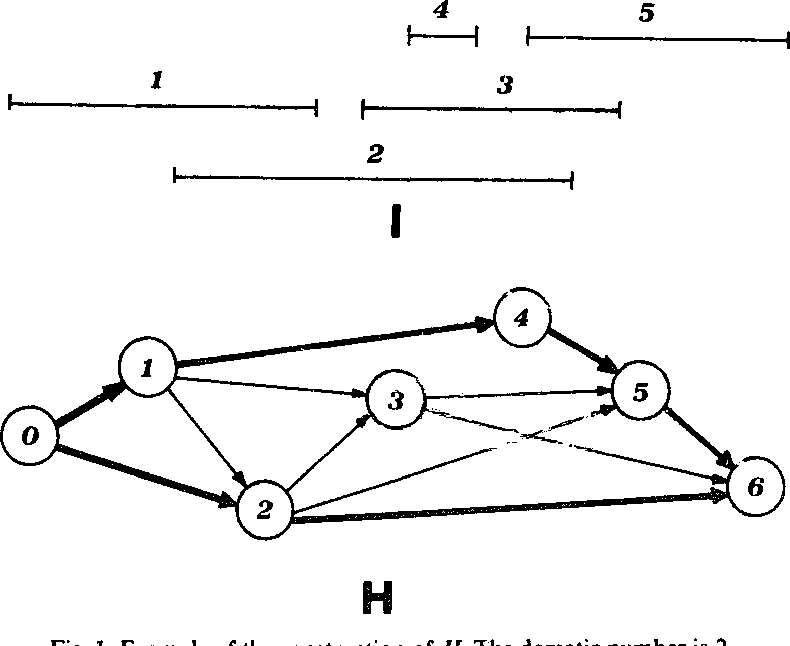•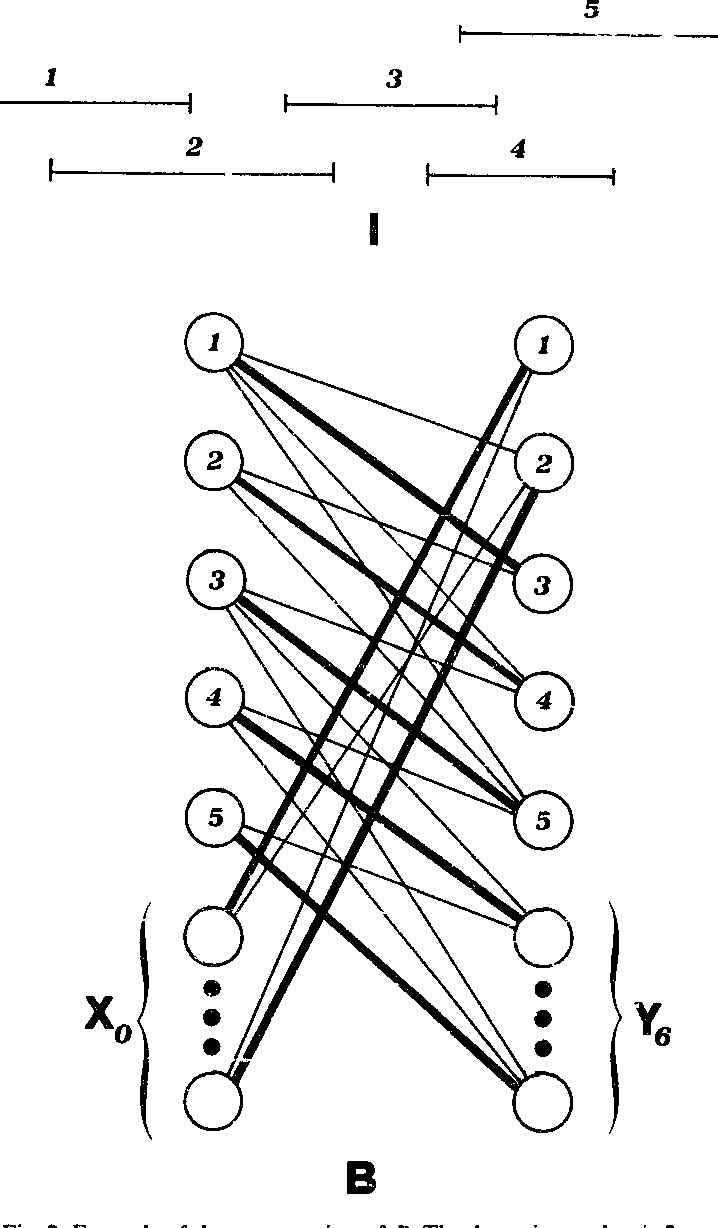Is this relevant?
1986
1986
All graphs considered in this paper are finite graphs without loops and multiple edges. The domatic number of a graph was defined… Expand
Is this relevant?